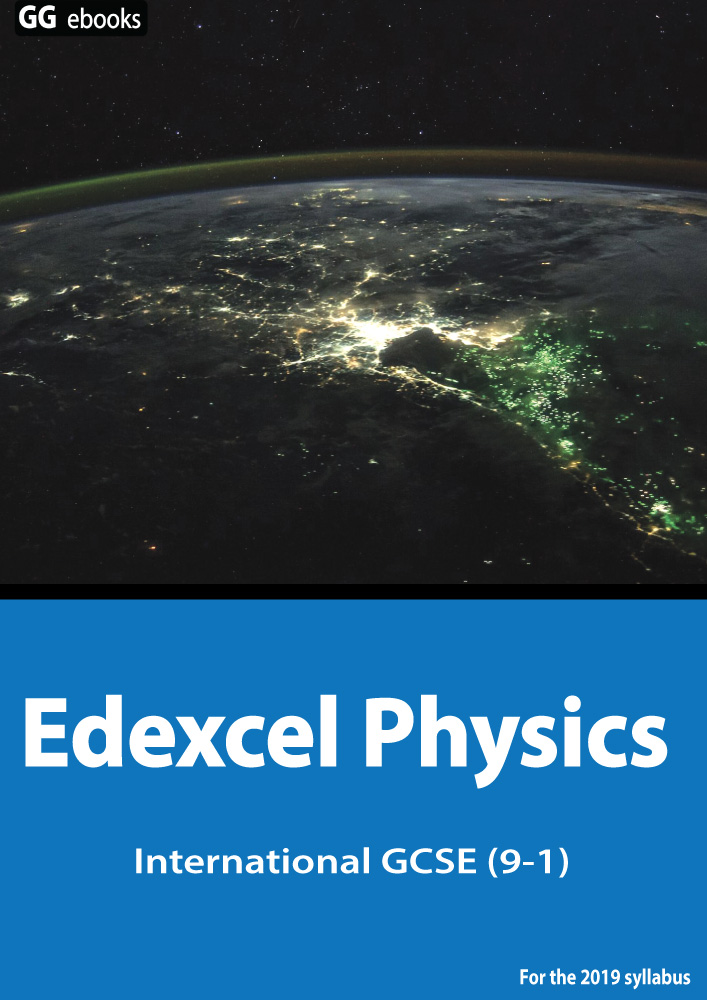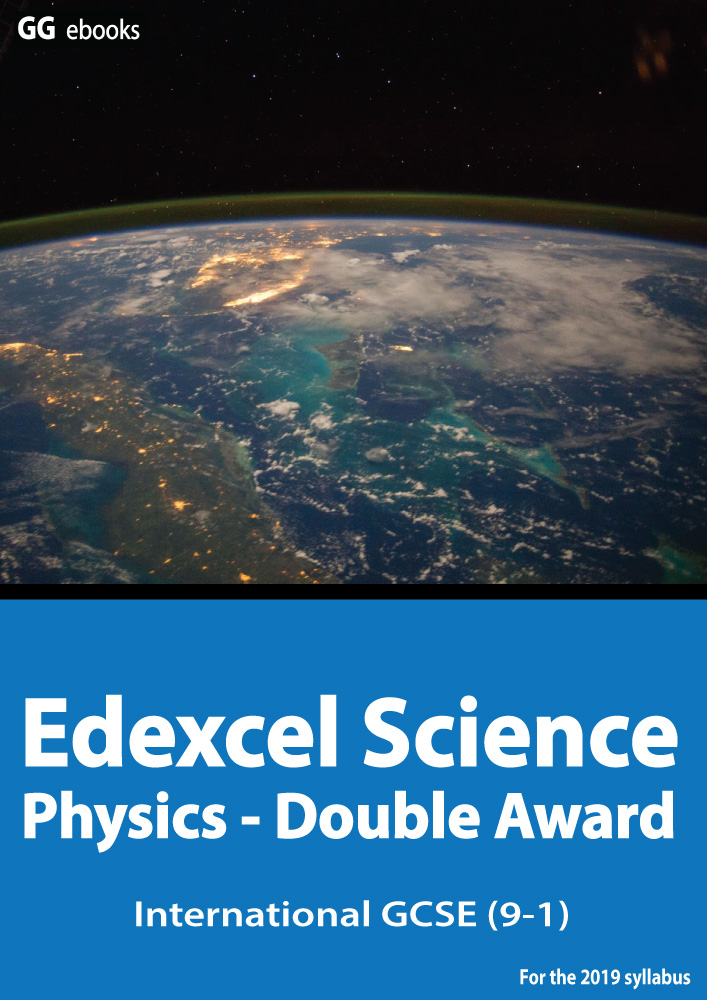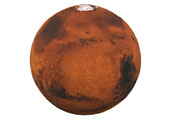Edexcel IGCSE - Forces B

Page 1

 NEED HELP? Edexcel iGCSE digital textbooks From the makers of GradeGorilla. GGebooks.com (Opens in a new tab)Weight, Stopping Distances, Hooke's Law, Vectors
1+2. Choose the correct units for the following measurements:
1. Mass
2. Weight

3. Which of these is NOT a type of force?

• A). Magnetic
• B). Electrostatic
• C). Acceleration
• D). Friction

4. Which of these sentences is correct?

• A). You are weightless on the moon
• B). Your mass changes as you travel into space
• C). Your weight is a measure of the density of your body
• D). Your weight is the pull of gravity on you.
On Mars the strength of gravity is 4 N / kg.5. What is the weight of a 60kg astronaut on Mars?

• A). 60N
• B). 240kg
• C). 15N
• D). 240N

6. What is the mass of a Mars rock of weight 2kN?

• A). 8 kg
• B). 8000N
• C). 50 N
• D). 500kg

7-11. A long wire is stretched using a large force. The table shows the length of the wire for different forces.

 Force (N) Length (m) 0 9.2 100 10.0 200 10.8 300 11.6 400 12.4 500 13.2Weight

7. What is the extension of the wire when the first 100N is applied?

8. What would be the extension if 50 N force were applied?
9. Hookes law states that the is proportional to the applied force.
10. What force would produce a length of 11.2 m?

11. 'Elastic behaviour' is best described as...

• A) the ability of a material to recover its original shape, once a force has been removed.
• B) the abililty of a material to stretch under a load.
• C) the abilty of a material to deform, bend or stretch.
• D) the ability of a material to produce a backward force once it is stretched.